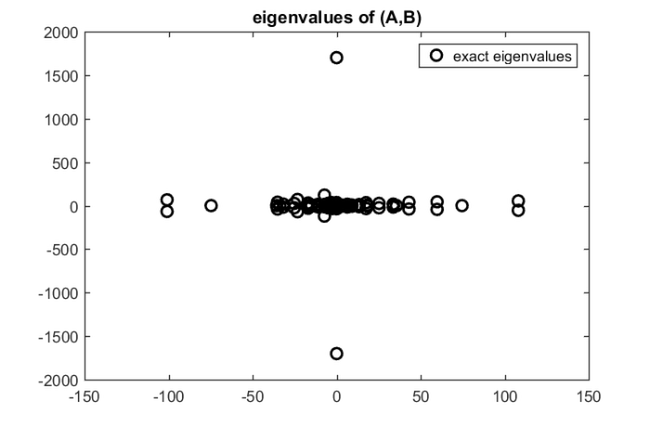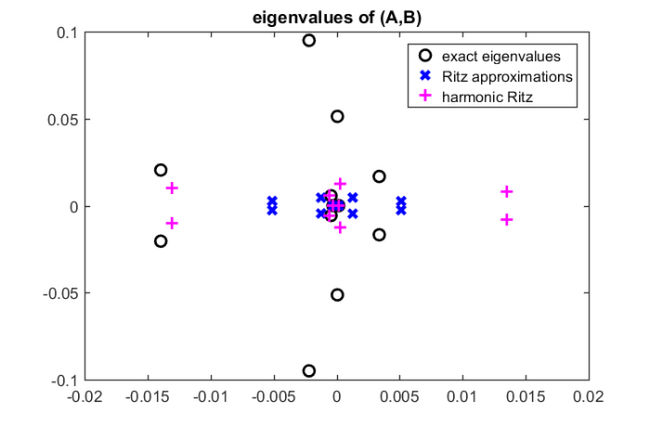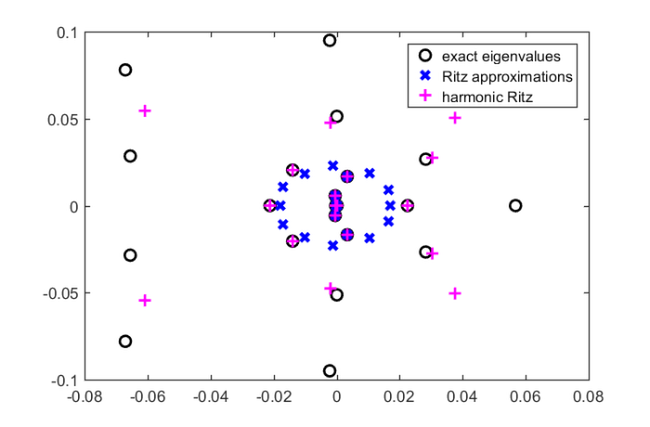# Solving large sparse eigenvalue problems

## Introduction

The first use of rational Krylov methods was for the solution of large sparse eigenvalue problems $A\mathbf x = \lambda B \mathbf x$, where $A$ and $B$ are $N\times N$ matrices and $(\lambda,\mathbf x)$ are the wanted eigenpairs; see [3, 4, 5, 6]. Let $\mathbf{b}$ be an $N\times 1$ vector and $m$ a positive integer. The rational Krylov space $\mathcal{Q}_{m+1}(A,\mathbf{b}, q_m)$ is defined as $\mathcal{Q}_{m+1}(A,\mathbf{b}, q_m) = q_m(A)^{-1} \mathrm{span} \{ \mathbf{b},A\mathbf{b},\ldots,A^m \mathbf{b}\}.$ Here, $q_m$ is a polynomial of degree at most $m$ having roots $\xi_1,\ldots,\xi_m$, called poles of the rational Krylov space. If $q_m$ is a constant nonzero polynomial, then $\mathcal{Q}_{m+1}(A,\mathbf{b}, q_m)$ is a standard (polynomial) Krylov space.

The rational Arnoldi method [4, 5] can be used to compute an orthonormal basis $V_{m+1}$ of $\mathcal{Q}_{m+1}$ such that a rational Arnoldi decomposition $A V_{m+1}\underline{K_m} = B V_{m+1} \underline{H_m}$ is satisfied, where $\underline{H_m}$ and $\underline{K_m}$ are upper Hessenberg matrices of size $(m+1)\times m$.

In the following we initialize two matrices for the pencil $(A, B)$ and plot the full spectrum $\Lambda(A, B)$. For simplicity we take $B=I$ and choose a rather small size to be able to compute all eigenvalues exactly.

load west0479
A = west0479;
B = speye(479);
ee = eig(full(A), full(B)); % Cannot do this for larger matrices!
figure, plot(ee, 'ko', 'linewidth', 2), hold on
title('eigenvalues of (A,B)')
legend('exact eigenvalues')We now construct a rational Krylov space with $m=10$ poles all set to zero, i.e., we are interested in the generalized eigenvalues of $(A,B)$ closest to zero. The inner product for the rational Krylov space can be defined by the user, or otherwise is the standard Euclidean one. (Since $B=I$ in this example, the two coincide.)

rng(0);
b  = randn(479,1);
m  = 10;
xi = repmat(0, 1, m);
param.inner_product = @(x,y) y'*B*x;
[V, K, H] = rat_krylov(A, B, b, xi, param);

warning off, nrmA = normest(A); nrmB = normest(B); warning on


We can easily check the validity of the rational Arnoldi decomposition by veryfing that the residual norm is close to machine precision:

disp(norm(A*V*K - B*V*H)/(nrmA + nrmB))

   2.6796e-18


The basis $V_{m+1}$ is close to orthonormal too:

disp(norm(param.inner_product(V, V)-eye(m+1)))

   7.5209e-16


## Extracting approximate eigenpairs

A common approach for extracting approximate eigenpairs from a search space is by using Ritz approximations or variants thereof. Let $C$ be an $N\times N$ matrix, and $X$ an $N\times m$ matrix. The pair $(\vartheta, \mathbf{y}\equiv X\mathbf{z})$ is called a

• Ritz pair for $C$ with respect to $\mathcal{R}(X)$ if $C\mathbf{y}-\vartheta \mathbf{y} \perp \mathcal{R}(X)$;
• harmonic Ritz pair for $C$ with respect to $\mathcal{R}(X)$ if $C\mathbf{y}-\vartheta \mathbf{y} \perp \mathcal{R}(CX)$.

Assume that $B$ is nonsingular. It follows easily (see [1, Lemma 2.4] and [2, Theorem 2.1]) from $A V_{m+1}\underline{K_m} = B V_{m+1} \underline{H_m}$ and the definition of (harmonic) Ritz pairs given above that

• Ritz pairs $(\vartheta, \mathbf{y}\equiv V_{m+1}\underline{K_m} \mathbf{z})$ for $B^{-1}A$ with respect to $\mathcal{R}(V_{m+1}\underline{K_m})$ arise from solutions of the generalized eigenvalue problem $\underline{K_m}^*\underline{H_m}^{\phantom{.}}\mathbf{z} = \vartheta \underline{K_m}^*\underline{K_m}^{\phantom{.}}\mathbf{z}$. Since $\underline{K_m}^{\phantom{.}}$ is of full rank, $\underline{K_m}^*\underline{K_m}^{\phantom{.}}$ is nonsingular, and we can equivalently solve the standard eigenvalue problem $\underline{K_m}^\dagger\underline{H_m}^{\phantom{.}}\mathbf{z} = \vartheta \mathbf{z}$;
• harmonic Ritz pairs $(\vartheta, \mathbf{y}\equiv V_{m+1}\underline{K_m} \mathbf{z})$ for $B^{-1}A$ with respect to $\mathcal{R}(V_{m+1}\underline{K_m})$ arise from solutions of the generalized eigenvalue problem $\underline{H_m}^*\underline{H_m}^{\phantom{.}}\mathbf{z} = \vartheta \underline{H_m}^*\underline{K_m}^{\phantom{.}}\mathbf{z}$. Since $\underline{H_m}^{\phantom{.}}$ is of full rank, $\underline{H_m}^*\underline{H_m}^{\phantom{.}}$ is nonsingular, and we can equivalently solve the standard eigenvalue problem $\underline{H_m}^\dagger\underline{K_m}^{\phantom{.}}\mathbf{z} = \lambda \mathbf{z}$, and take $\vartheta = \frac{1}{\lambda}$.
[Xr, Dr] = eig(K\H);
[Xh, Dh] = eig(H\K);
ritz = diag(Dr);
hrm_ritz = 1./diag(Dh);

plot(real(ritz), imag(ritz),'bx', 'linewidth', 3)
plot(real(hrm_ritz), imag(hrm_ritz),'m+', 'linewidth', 2)
axis([-0.02, 0.02, -0.1, 0.1])
legend('exact eigenvalues', ...
'Ritz approximations', ...
'harmonic Ritz')## Accuracy of the approximate eigenpairs

We can evaluate the accuracy of the (harmonic) Ritz pairs from the relative residual norm $\frac{\|A\mathbf{y}-\vartheta B \mathbf{y}\|_2}{(\|A\|_2+|\vartheta|\|B\|_2)\|\mathbf{y}\|_2}$. From the rational Arnoldi decomposition $A V_{m+1}\underline{K_m} = B V_{m+1} \underline{H_m}$ we have $A\mathbf{y}-\vartheta B\mathbf{y} = AV_{m+1}\underline{K_m} \mathbf{z} - \vartheta BV_{m+1}\underline{K_m} \mathbf{z} = BV_{m+1}(\underline{H_m}-\vartheta\underline{K_m})\mathbf{z}$. Hence, a cheap estimate of the accuracy of an approximate eigenpair is the norm $\|(\underline{H_m}-\vartheta\underline{K_m})\mathbf{z}\|_2$. If this norm is small compared to $\|B^{-1}A\|_2$, we have computed an eigenpair of a nearby problem. It seems that in this example two eigenpairs have already converged to very high accuracy:

approx_residue = @(X) arrayfun(@(i)norm(X(:, i)), 1:size(X, 2));

approx_res = [approx_residue(H*Xr-K*Xr*Dr).' ...
approx_residue(H*Xh-K*Xh*diag(hrm_ritz)).'];
disp(approx_res)

   5.1976e-08   4.5063e-19
5.1976e-08   6.1961e-17
4.5828e-08   8.2255e-07
4.5828e-08   8.2255e-07
6.6227e-08   1.5529e-07
6.6227e-08   1.5529e-07
6.6756e-08   1.5303e-07
6.6756e-08   1.5303e-07
1.2580e-17   2.3565e-07
1.5728e-15   2.3565e-07


## Expanding the rational Arnoldi decomposition

Let us perform $8$ further iterations with rat_krylov, with $2$ repeated poles being the harmonic Ritz eigenvalues expected to converge next. Since the poles appear in complex-conjugate pairs, we can turn on the real flag for rat_krylov and end up with a real-valued quasi rational Arnoldi decomposition .

[~, ind] = sort(approx_res(:, 2));
xi = repmat(hrm_ritz(ind([3, 4]))', 1, 4);
param.real = 1;

[V, K, H] = rat_krylov(A, B, V, K, H, xi, param);


Let us check the residual norm of the extended rational Arnoldi decomposition, and verify that the decomposition has the original $10$ poles at zero, and the newly selected $8$ poles.

disp(norm(A*V*K - B*V*H)/(nrmA + nrmB))
disp(util_pencil_poles(K, H).')

   2.9720e-18
0.0000e+00 + 0.0000e+00i
0.0000e+00 + 0.0000e+00i
0.0000e+00 + 0.0000e+00i
0.0000e+00 + 0.0000e+00i
0.0000e+00 + 0.0000e+00i
0.0000e+00 + 0.0000e+00i
0.0000e+00 + 0.0000e+00i
0.0000e+00 + 0.0000e+00i
0.0000e+00 + 0.0000e+00i
0.0000e+00 + 0.0000e+00i
2.8261e-04 - 1.2537e-02i
2.8261e-04 + 1.2537e-02i
2.8261e-04 - 1.2537e-02i
2.8261e-04 + 1.2537e-02i
2.8261e-04 - 1.2537e-02i
2.8261e-04 + 1.2537e-02i
2.8261e-04 - 1.2537e-02i
2.8261e-04 + 1.2537e-02i


Finally, the (improved) $18$ Ritz pairs are evaluated, both standard and harmonic.

[Xr, Dr] = eig(K\H);
[Xh, Dh] = eig(H\K);

ritz = diag(Dr);
hrm_ritz = 1./diag(Dh);

approx_res = [approx_residue(H*Xr-K*Xr*Dr).' ...
approx_residue(H*Xh-K*Xh*diag(hrm_ritz)).'];

disp(approx_res)

   7.8102e-10   8.8386e-20
7.8102e-10   2.5844e-19
1.0521e-09   1.2433e-13
1.5917e-08   1.2433e-13
3.4226e-10   3.3683e-11
3.4226e-10   2.3079e-10
5.3472e-10   3.5878e-13
5.3472e-10   3.5878e-13
1.1481e-09   2.3904e-11
1.1481e-09   2.3904e-11
3.9385e-09   9.2915e-09
3.9385e-09   9.2915e-09
6.0424e-16   4.0670e-09
2.5439e-11   4.0670e-09
2.5439e-11   3.3345e-09
9.7244e-12   3.3345e-09
9.7244e-12   9.9538e-09
4.4158e-16   9.9538e-09

figure, plot(ee, 'ko', 'linewidth', 2), hold on
plot(real(ritz), imag(ritz),'bx', 'linewidth', 3)
plot(real(hrm_ritz), imag(hrm_ritz),'m+', 'linewidth', 2)
axis([-0.08, 0.08, -0.1, 0.1])
legend('exact eigenvalues', ...
'Ritz approximations', ...
'harmonic Ritz')Harmonic Ritz pairs are typically better than (standard) Ritz pairs for interior eigenvalues, thought this is not yet fully understood. Also, for symmetric matrices the two sets of Ritz values interlace each other, and hence their distance is not large as ultimately both sets converge to the same eigenvalues.

## References

 G. De Samblanx and A. Bultheel. Using implicitly filtered RKS for generalised eigenvalue problems, J. Comput. Appl. Math., 107(2):195--218, 1999.

 R. B. Lehoucq and K. Meerbergen. Using generalized Cayley transformations within an inexact rational Krylov sequence method, SIAM J. Matrix Anal. Appl., 20(1):131--148, 1998.

 A. Ruhe. Rational Krylov sequence methods for eigenvalue computation, Linear Algebra Appl., 58:391--405, 1984.

 A. Ruhe. Rational Krylov algorithms for nonsymmetric eigenvalue problems, Recent Advances in Iterative Methods. Springer New York, pp. 149--164, 1994.

 A. Ruhe. Rational Krylov: A practical algorithm for large sparse nonsymmetric matrix pencils, SIAM J. Sci. Comput., 19(5):1535--1551, 1998.

 A. Ruhe. The rational Krylov algorithm for nonsymmetric eigenvalue problems. III: Complex shifts for real matrices, BIT, 34(1):165--176, 1994.